ShellSort

• Difficulty Level : Medium
• Last Updated : 17 Aug, 2021

ShellSort is mainly a variation of Insertion Sort. In insertion sort, we move elements only one position ahead. When an element has to be moved far ahead, many movements are involved. The idea of shellSort is to allow exchange of far items. In shellSort, we make the array h-sorted for a large value of h. We keep reducing the value of h until it becomes 1. An array is said to be h-sorted if all sublists of every h’th element is sorted.

Following is the implementation of ShellSort.

C++

 // C++ implementation of Shell Sort#include  using namespace std; /* function to sort arr using shellSort */int shellSort(int arr[], int n){    // Start with a big gap, then reduce the gap    for (int gap = n/2; gap > 0; gap /= 2)    {        // Do a gapped insertion sort for this gap size.        // The first gap elements a[0..gap-1] are already in gapped order        // keep adding one more element until the entire array is        // gap sorted        for (int i = gap; i < n; i += 1)        {            // add a[i] to the elements that have been gap sorted            // save a[i] in temp and make a hole at position i            int temp = arr[i];             // shift earlier gap-sorted elements up until the correct            // location for a[i] is found            int j;                       for (j = i; j >= gap && arr[j - gap] > temp; j -= gap)                arr[j] = arr[j - gap];                         //  put temp (the original a[i]) in its correct location            arr[j] = temp;        }    }    return 0;} void printArray(int arr[], int n){    for (int i=0; i

Java

 // Java implementation of ShellSortclass ShellSort{    /* An utility function to print array of size n*/    static void printArray(int arr[])    {        int n = arr.length;        for (int i=0; i 0; gap /= 2)        {            // Do a gapped insertion sort for this gap size.            // The first gap elements a[0..gap-1] are already            // in gapped order keep adding one more element            // until the entire array is gap sorted            for (int i = gap; i < n; i += 1)            {                // add a[i] to the elements that have been gap                // sorted save a[i] in temp and make a hole at                // position i                int temp = arr[i];                 // shift earlier gap-sorted elements up until                // the correct location for a[i] is found                int j;                for (j = i; j >= gap && arr[j - gap] > temp; j -= gap)                    arr[j] = arr[j - gap];                 // put temp (the original a[i]) in its correct                // location                arr[j] = temp;            }        }        return 0;    }     // Driver method    public static void main(String args[])    {        int arr[] = {12, 34, 54, 2, 3};        System.out.println("Array before sorting");        printArray(arr);         ShellSort ob = new ShellSort();        ob.sort(arr);         System.out.println("Array after sorting");        printArray(arr);    }}/*This code is contributed by Rajat Mishra */

Python3

 # Python3 program for implementation of Shell Sort def shellSort(arr):    gap = len(arr) // 2 # initialize the gap     while gap > 0:        i = 0        j = gap                 # check the array in from left to right        # till the last possible index of j        while j < len(arr):                 if arr[i] >arr[j]:                arr[i],arr[j] = arr[j],arr[i]                         i += 1            j += 1                     # now, we look back from ith index to the left            # we swap the values which are not in the right order.            k = i            while k - gap > -1:                 if arr[k - gap] > arr[k]:                    arr[k-gap],arr[k] = arr[k],arr[k-gap]                k -= 1         gap //= 2  # driver to check the codearr2 = [12, 34, 54, 2, 3]print("input array:",arr2) shellSort(arr2)print("sorted array",arr2) # This code is contributed by Shubham Prashar (SirPrashar)

C#

 // C# implementation of ShellSortusing System; class ShellSort{    /* An utility function to       print array of size n*/    static void printArray(int []arr)    {        int n = arr.Length;        for (int i=0; i 0; gap /= 2)        {            // Do a gapped insertion sort for this gap size.            // The first gap elements a[0..gap-1] are already            // in gapped order keep adding one more element            // until the entire array is gap sorted            for (int i = gap; i < n; i += 1)            {                // add a[i] to the elements that have                // been gap sorted save a[i] in temp and                // make a hole at position i                int temp = arr[i];                 // shift earlier gap-sorted elements up until                // the correct location for a[i] is found                int j;                for (j = i; j >= gap && arr[j - gap] > temp; j -= gap)                    arr[j] = arr[j - gap];                 // put temp (the original a[i])                // in its correct location                arr[j] = temp;            }        }        return 0;    }     // Driver method    public static void Main()    {        int []arr = {12, 34, 54, 2, 3};        Console.Write("Array before sorting :\n");        printArray(arr);         ShellSort ob = new ShellSort();        ob.sort(arr);         Console.Write("Array after sorting :\n");        printArray(arr);    }} // This code is contributed by nitin mittal.

Javascript



Output:

Array before sorting:
12 34 54 2 3
Array after sorting:
2 3 12 34 54

Time Complexity: Time complexity of above implementation of shellsort is O(n2). In the above implementation gap is reduce by half in every iteration. There are many other ways to reduce gap which lead to better time complexity. See this for more details.

Snapshots: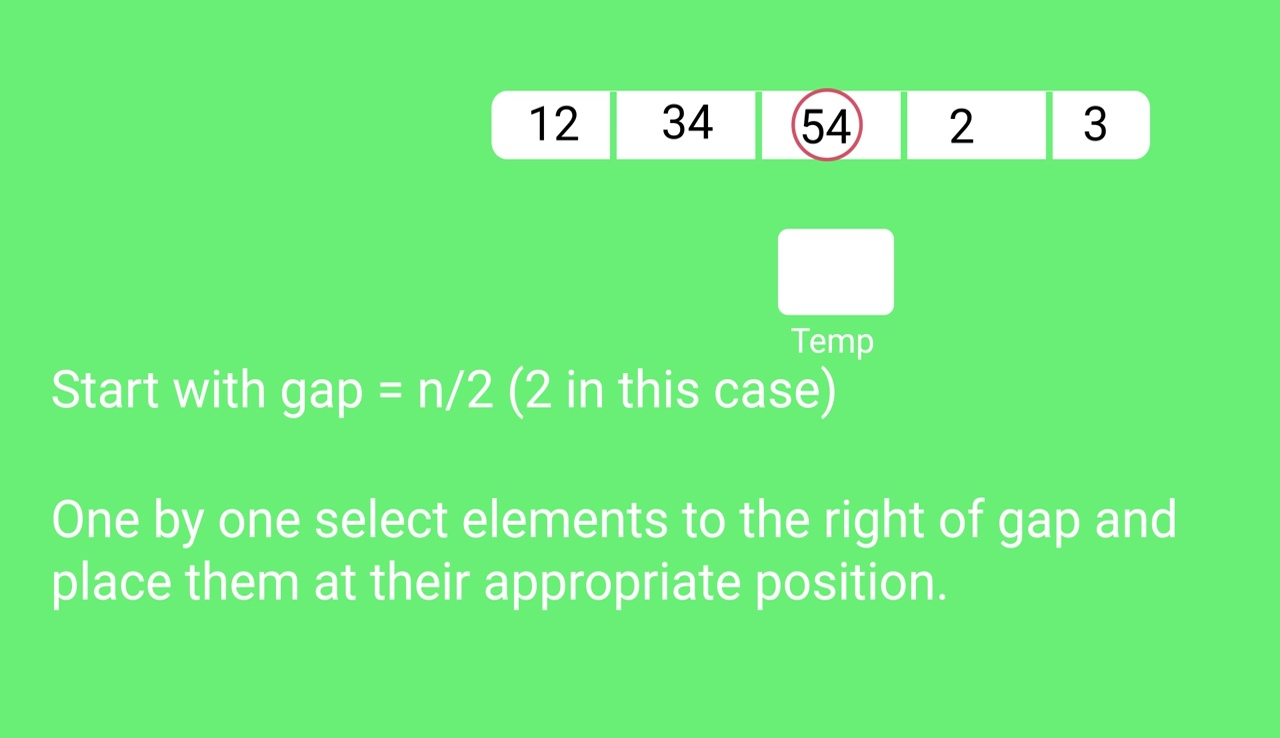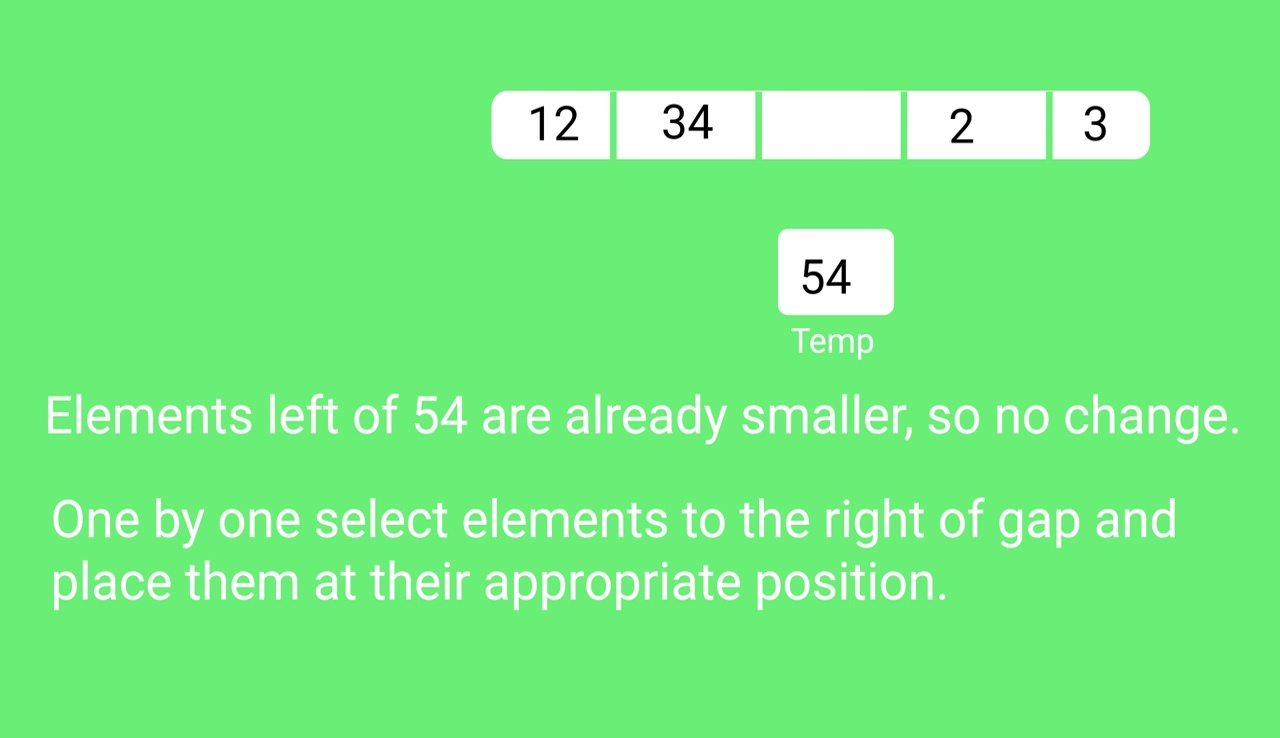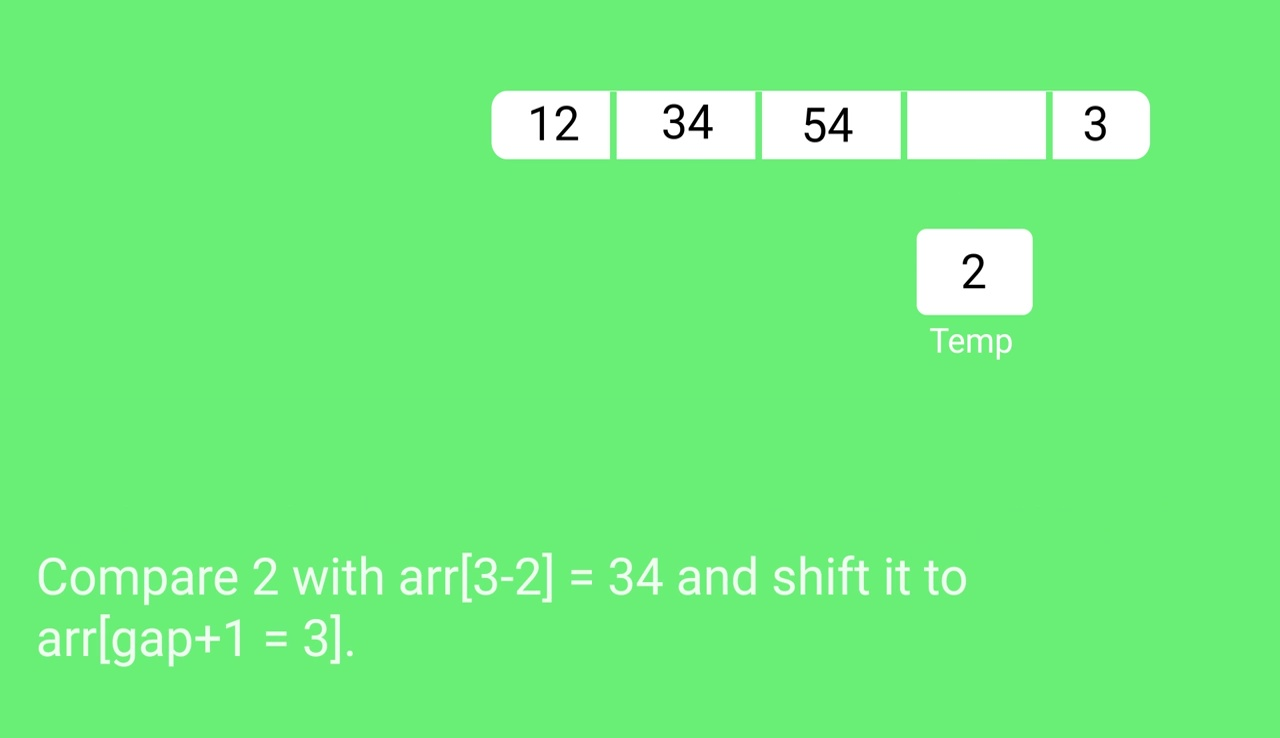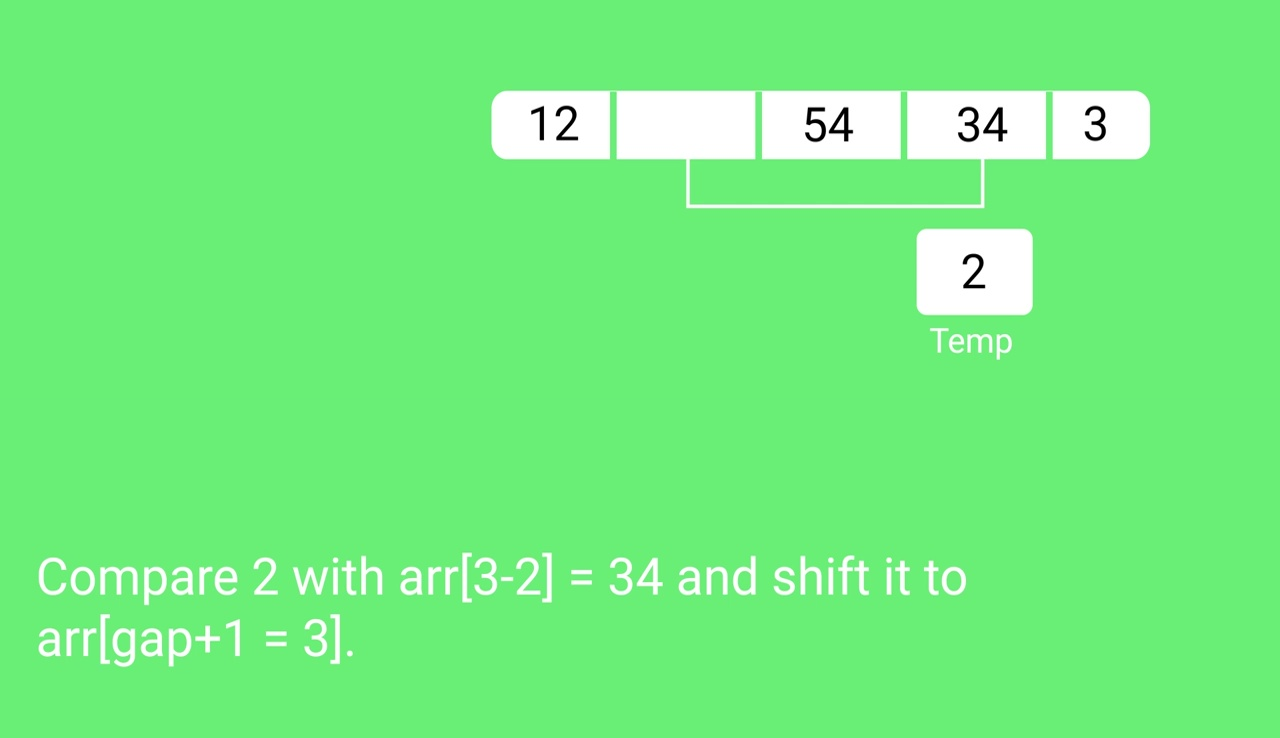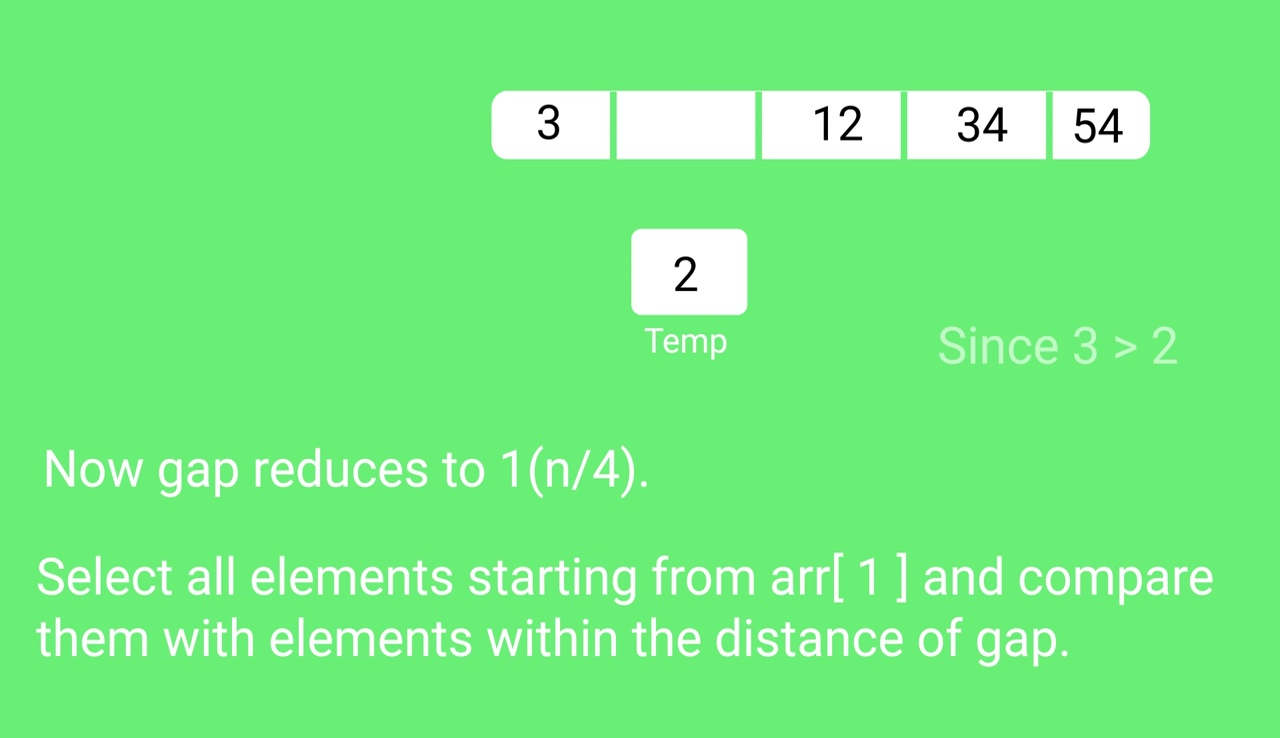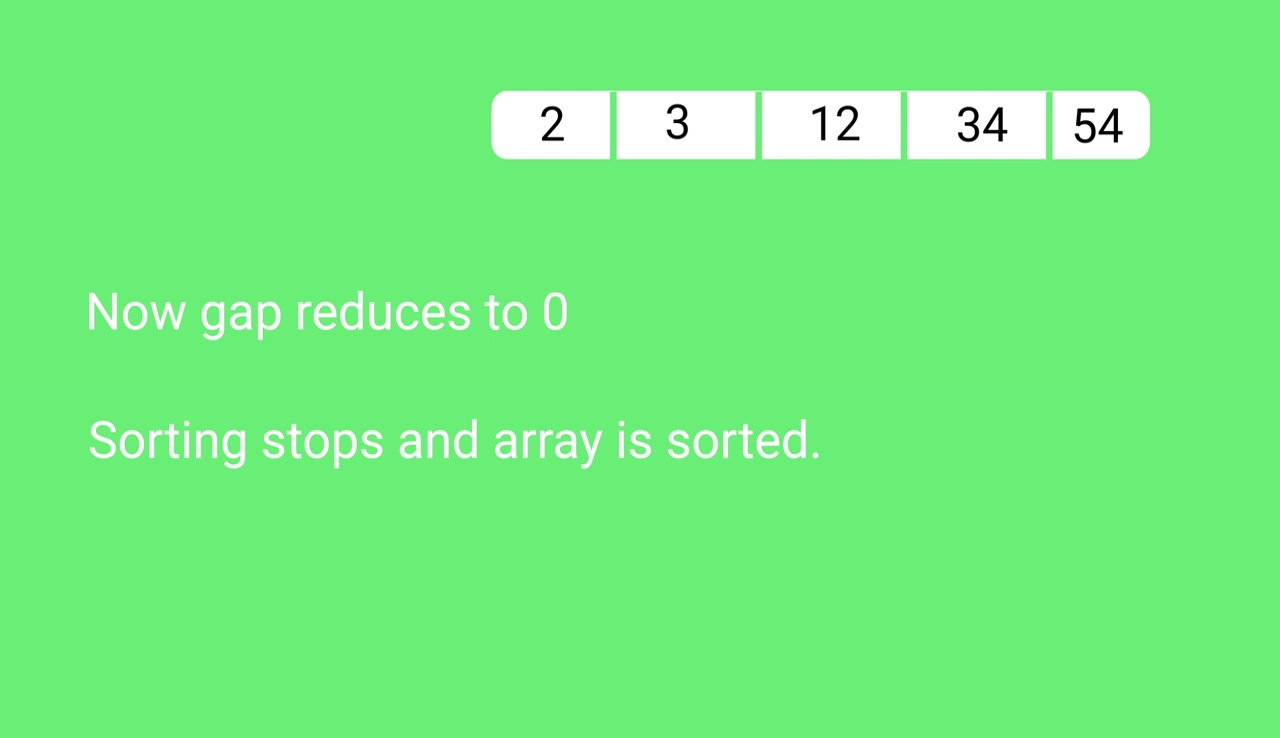Quiz on Shell Sort

Other Sorting Algorithms on GeeksforGeeks/GeeksQuiz:

My Personal Notes arrow_drop_up